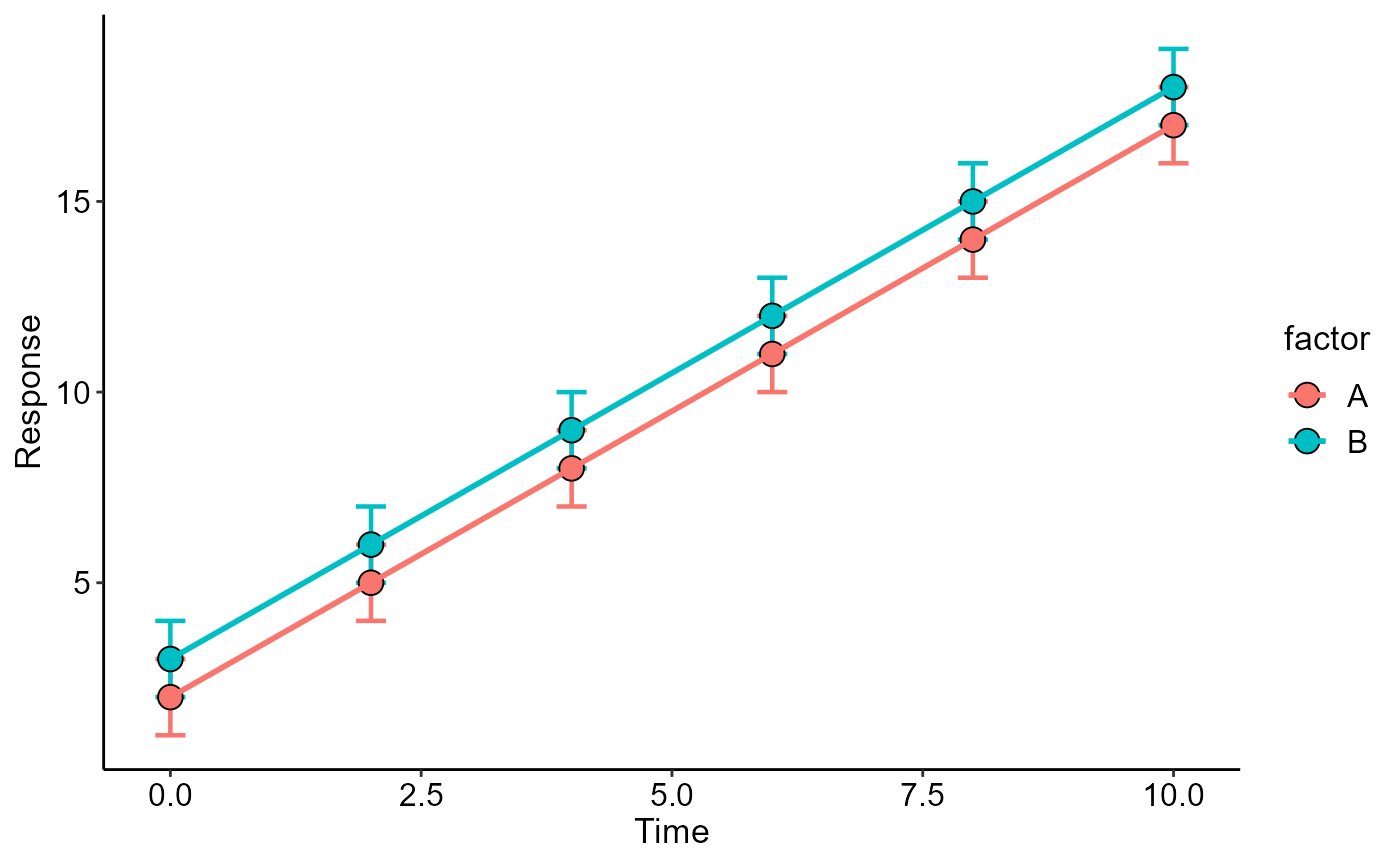Performs a descriptive line graph with standard deviation bars

line_plot(
time,
response,
factor = NA,
errorbar = "sd",
ylab = "Response",
xlab = "Time",
legend.position = "right",
theme = theme_classic()
)

## Arguments

time

Vector containing the x-axis values

response

Vector containing the y-axis values

factor

Vector containing a categorical factor

errorbar

Error bars (sd or se)

ylab

y axis title

xlab

x axis title

legend.position

Legend position

theme

ggplot2 theme (default is theme_classic())

## Value

Returns a line chart with error bars

## Author

Gabriel Danilo Shimizu, shimizu@uel.br

Leandro Simoes Azeredo Goncalves

Rodrigo Yudi Palhaci Marubayashi

## Examples

dose=rep(c(0,2,4,6,8,10),e=3,2)
resp=c(seq(1,18,1),seq(2,19,1))
fator=rep(c("A","B"),e=18)
line_plot(dose,resp,fator)